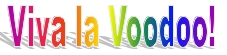Faqs | Help | @ | Contact |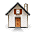## The Mighty Maximin and worst-case analysis

Nukes 6of6: Worst Case Scenario and Nuclear Waste Storage

This is one of my more recent campaigns. Indeed, it is a mini-campaign in its own right. Still, this particular project has an affinity with my Solution-Method-Free-Modeling campaign in that, both projects are concerned with the role of mathematical modeling in decision making. I might also add that this campaign inspired my Voodoo Decision-Making campaign.

The direct trigger for this project was the realization that there is something suspect in the interpretation that naive, and not so naive, users of Info-Gap Decision Theory — a purportedly new theory for decision making under severe uncertainty — give this theory.

In any case, the objective of this campaign is to clarify conceptual and technical issues associated with the modeling aspects of the classical Maximin paradigm, especially in the context of decision-making in the face of severe uncertainty.

## Overview

However incomprehensible this may appear to seasoned analysts/scholars working in the area of decision-making under uncertainty, there are those who profess to be experts in the field yet they are not conversant with Worst-Case Analysis and Wald's Maximin Principle. This can range from ignorance about the existence of these two central modeling tools, to a shaky grasp of their role and place in decision theory, to a total lack of understanding of the mathematical modeling issues that come into play in the application of these important modeling tools.

So here are some thoughts on this topic.

The basic idea underlying the Maximin paradigm can be summarized as follows:

 Maximin Rule Rank alternatives by their worst possible outcomes: adopt the alternative the worst outcome of which is at least as good as the worst outcome of the other alternatives.

This paradigm was conceived by the mathematician Abraham Wald (1902-1950). It is in fact an adaptation of John von Neumann's (1903-1957) Maximin paradigm that he developed in the context of game theory. Wald hit on the idea of adapting this paradigm to the framework of decision-making under uncertainty thus casting Uncertainty (call it Nature) as the player pit against the decision maker. The idea here is that, to play it safe, the decision maker assumes that Nature constitutes an antagonistic adversary.

So, if the decision maker maximizes her "utility", Nature will attampt to minimze the "utility", whereas if the decision maker minimizes the "utility", Nature will attempt to maximize the "utility". The first case is captured by the term Maximin and the second case by the term Minimax.

The Maximin and Minimax models are essentially equivalent (subject to multiplying the "utility" by -1) and the choice between them is a matter of convenience.

Note that this dark view of uncertainty is not meant to be taken as a philosophical statement about the nature of reality. Rather, the point of the Maximin/Minimax model is that Nature is used as a device for expressing the decision maker's attitude towards the risks associated with uncertainty. So, like my dear wife, in this framework the decision maker takes an extremely pessimistic stance by assuming that the "worst-case-scenario" pertaining to her decision will be realized.

Not surprisingly, therefore, Maximin/Minimax has become almost synonymous with robust decision-making not only in classical decision theory but in other areas as well. For instance, here is the abstract of the entry Robust Control by Noah Williams in the New Palgrave Dictionary of Economics, Second Edition, 2008:

 Robust control is an approach for confronting model uncertainty in decision making, aiming at finding decision rules which perform well across a range of alternative models. This typically leads to a minimax approach, where the robust decision rule minimizes the worst-case outcome from the possible set. This article discusses the rationale for robust decisions, the background literature in control theory, and different approaches which have been used in economics, including the most prominent approach due to Hansen and Sargent.

The following quote is from the book Robust Statistics by Huber (1981, pp. 16-17):

 But as we defined robustness to mean insensitivity with regard to small deviations from assumptions, any quantitative measure of robustness must somehow be concerned with the maximum degradation of performance possible for an e-deviation from the assumptions. The optimally robust procedure minimizes this degradation and hence will be a minimax procedure of some kind.

Keeping in mind that "minimax" signifies the reverse of Maximin, in this discussion, we are concerned for the most part, with the Maximin option.

## Math formulation

The two most prevalent (equivalent) formal mathematical formulations of the Maximin paradigm are: the classic formulation and the mathematical programming (MP) formulation. Here they are side by side:where the double-lined R denotes the real line.

Note that an instance of a Maximin model is specified by a triplet (D,S,f) whose elements can be interpreted as follows:

• D is a set, called Decision Space.
It contains the decisions available to the decision maker.

• S(d) is the set of states associated with decision d.
Formally the union of S(d) over all d in D is called the state space. In the context of decision-making under uncertainty, the states are determined, managed and controlled by Nature.

• f = f(d,s) is the objective function that the decision maker seeks to maximize (over d in D)

In short, these formulations describe a game played by two players: one player (the decision maker) tries to maximize the objective function by controlling d in D, while the other player (Nature) tries to minimize the objective function by controlling s in S(d). Observe that when Nature decides on her best s she knows the value of d selected by the decision maker. In plain language, in this game the decision maker plays first whereupon Nature responds to his choice of a d in D.

In cases where robustness is sought with respect to constraints, rather than "utility", the Maximin model would be formulated as follows: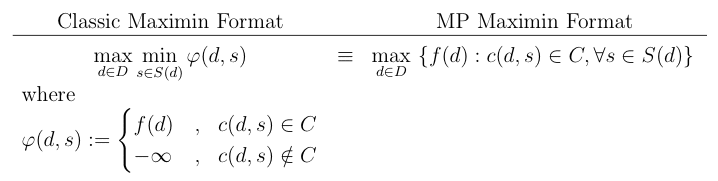where — without loss of generality — the constraint requires the value of c(d,s) to be an element of some given set C. In other words, here the decision maker's goal is to maximize the utility f(d) over all d in D subject to the condition that c(d,s) is in C for all s in S(d). For her part, Nature will attempt to violate this constraint — if possible — by a proper choice of s in S(d).

And if robustness is sought with repsect to the objective function and the constraint, the Maximin model would be formulated as follows:The Minimax formulations are similar except that the min and max operations are interchanged. In this case the decision maker seeks to minimize the objective function and therefore antagonistic Nature attempts to maximize it.

For our purposes here, the above discussion is sufficient. We need point out, however, that Wald's Maximin model plays a key role in classical decision theory, robust optimization, economics, statistics, and control theory.

To some this paradigm is second nature, to others it is a puzzle.

### Remark:

The fact that a "max" and a "min" appear in a formulation of a decision-making model does not automatically render the model a Maximin model. For example, consider the following decision-making model: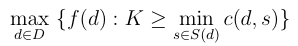In general, this is not a Maximin model because we do not have here a situation where Nature (deciding on s) "antagonizes" the decision maker (deciding on d). Or, in other words, the idea here is not to identify the "best worst-case".

This is so, because here not all s in S(d) are required to satisfy the constraint K ≥ c(d,s). So, by selecting s to minimize c(d,s) over s in S(d), Nature does not select the worst s in S(d) with regard to the constraint K ≥ c(d,s), which means that some s in S(d) are "allowed" to violate the constraint K ≥ c(d,s).

In a word, all that is required here is that "at least one" element of S(d) satisfy the constraint K ≥ c(d,s).

This, as a matter of fact is a Maximax model, observing thatwhereNote that in this framework Nature is not antagonistic towards the decision maker. To the contrary, here Nature cooperates with her.

In contrast, the modelis a Maximin model even though no "min" occurs in the formulation. Note that here all s in S(d) must satisfy the constraint K ≥ c(d,s). Thus,where## Variations on the same theme

Now, the pessimistic stance to uncertainty prescribed by Wald's Maximin paradigm proves far too conservative for many applications. Not surprisingly, therefore, a number of attempts have been made to modify the paradigm's grim approach to uncertainty with the view to mitigate its excessive conservatism.

• Hurwicz' Optimism-Pessimism Model (1950):
The mathematician/economist Leonid Hurwicz (1917-2008) put forward a model that seeks to strike a balance between two polar approaches to uncertainty: Wald's pessimistic view and a view of Nature as a cooperative player. The idea here is to seek the best "weighted sum" of these two approaches. So, the application of this model requires specifying the decision maker's Optimism - Pessimism index. The value of this index can range from zero (extreme optimism) to one (extreme pessimism) and is used to determine the weighted sum of the optimistic and pessimistic outcomes.

• Savage's Minimax regret model (1951):
Of course, some people fret more about "regrets" than about "payoffs" or "losses". I for one can testify to the validity of this observation, citing my dear wife as a perfect example.

In classical decision theory the regret associated with a decision is the difference between the actual payoff generated by the decision and the optimal payoff generated by a decision that is best with respect to the assumed state of Nature. This concept was introduced by the mathematician/statistician Leonard J. Savage (1917-1971) again, with the view to mitigate the extreme pessimism of Wald's Maximin paradigm.

Savage's model (1951) prescribes that the decision-maker rank the decisions by applying a Minimax rule to "regrets" rather than "payoffs", where formally the regrets, r(d,s), are derived from the payoffs, f(d,s), yielded by the conventional Maximin model, as follows:

r(d,s) = f*(s) - f(d,s)

where f*(s) = max{f(x,s): x∈D}, that is, f*(s) is the best (over all decisions) payoff for a given state s.

The reader is advised that modeling the Maximin can involve a significant effort. Indeed, stating the paradigm in terms of a particular situation can often require of the modeler/analyst considerable insight, imagination and invention (see my paper The Mighty Maximin!).

## Decision Tables

In cases where the decision and state spaces are finite sets, the Maximin model can be depicted as a Decision Table. The convention is that the rows of the table represent decisions, the columns represent states and the entries of the table represent the rewards r(d,s), say in AU\$.

For example, consider the following simple decision table:

 s1 s2 s3 s4 d1 7 5 6 9 d2 4 8 6 5 d3 9 1 7 8

We now append an additional column to the table to list the security levels of the decisions. The security level of decision dj is the smallest reward in the j-th row of the table. This means that if we select decision dj, then no matter what state is realized, the actual reward will be no smaller than the security level.

So here is our decision table with the associated security levels (AU\$):

 s1 s2 s3 s4 SL(j) d1 7 5 6 9 5 d2 4 8 6 5 4 d3 9 1 7 8 1

What is left then is to find the largest security level. We indicate this in the usual/unusual way:

 s1 s2 s3 s4 SL(j) d1 7 5 6 9 5 ♥ d2 4 8 6 5 4 d3 9 1 7 8 1

In short, the Maximin rule selects decision d1. The optimal (maximal) security level is AU\$5.

## Bayes Rule vs Maximin Rule

Recently, I was greatly surprised to learn that apparently, there is a perception out there, that a Maximin decision rule can be simulated by a degenerate Bayes Rule that selects (with certainty) one of the states.

It is important therefore to set the record straight on this matter by showing that this is not so.

Recall that in the framework of Bayes Rule, some probability distribution function is assumed to operate on the state space and the performance of a decision, say d, is measured by the expected value of the reward r(d,s) determined by this distribution. Thus let E[d] denote the expected value of r(d,s) generated by decision d and the assumed probability distribution function of s.

In keeping with Bayes rule, decisions are ranked by their E[d] values — the larger the better. So the optimal decision according to this rule is one that maximizes E[d] over all the feasible values of d.

For example, consider the following (familiar) decision table:

 s1 s2 s3 s4 d1 7 5 6 1 d2 4 8 6 5 d3 9 1 7 8

To illustrate Bayes Rule in action we have to postulate a probability distribution over the four states. Suppose that we consider the vector p=(0.1, 0.2,0.4,0.3) for this purpose. The convention is to list these probabilities above the states:

 p(i) 0.1 0.2 0.4 0.3 s1 s2 s3 s4 d1 7 5 6 1 d2 4 8 6 5 d3 9 1 7 8

We now append an additional column to the table where we list the value of E[dj], j=1,2,3.

 p(i) 0.1 0.2 0.4 0.3 s1 s2 s3 s4 E[dj] d1 7 5 6 1 4.4 d2 4 8 6 5 5.8 d3 9 1 7 8 6.3

Thus, the best decision according to Bayes Rule is d3. It yields an expected reward of 6.3.

 p(i) 0.1 0.2 0.4 0.3 s1 s2 s3 s4 E[dj] d1 7 5 6 1 4.4 d2 4 8 6 5 5.8 d3 9 1 7 8 6.3 ♥

The point to note then is that here, all the decisions are evaluated in accordance with the same assumed probability distribution (over the state space). But in the framework of the Maximin model, each individual decision is evaluated on the basis of the worst case (state) pertaining to this decision alone. This means that, in general, there is no guarantee that a single probability distribution over the state space will be able to simulate the Maximin Rule.

If you remain unconvinced, consider the following simple case: there are two states, say s1 and s2, and three decisions, call them d1 , d2, and d3. Suppose that the reward table is as follows:

 s1 s2 d1 7 5 d2 4 8 d3 9 1

Appending the security levels column we have:

 s1 s2 SL(j) d1 7 5 5 ♥ d2 4 8 4 d3 9 1 1

We conclude then that the optimal decision prescribed by Maximin is d1, yielding the maximum security level of AU\$5.

In short, in this case the Maximin decision rule yields the following result:

Optimal decision: d1
Guaranteed reward: AU\$5

Note that there is no degenerate distribution on S = {s1,s2} that can simulate this result under Bayes Rule. If Nature selects s1 with probability 1, then clearly decision d3 is better than decision d1. If Nature selects s2 with probability 1, then clearly d2 is better than d1.

The two decision tables are as follows:

 p(i) 1 0 s1 s2 E[dj] d1 7 5 7 d2 4 8 4 d3 9 1 9 ♥

 p(i) 0 1 s1 s2 E[dj] d1 7 5 5 d2 4 8 8 ♥ d3 9 1 1

In short, in this case there is no degenerate distribution on the state space that can force Bayes Rule to select d1.

The conclusion is therefore that the Maximin Rule is not an instance of Bayes Rule. This, however, does not mean that the two rules are rivals. Rather, what this means is that, they complement one another.

So the moral of the story is that both are essential. Indeed, don't leave home without either of them. It is best to have both. Even if you travel to Australia to take up the best job in the world!

That said, we must not neglect to mention in this context another pillar of classical decision theory:

## Laplace's Principle of Insufficient Reason

This Principle — attributed to the French mathematician and astronomer Pierre-Simon, marquis de Laplace (1749 - 1827) — argues, by symmetry, that if there are n > 1 mutually exclusive and collectively exhaustive possibilities, then each possibility should be assigned the same probability (equal to 1/n).

To put it in more general terms, this Principle, which is also known as the Indifference Principle — so named by the British economist John Maynard Keynes, 1st Baron Keynes (1883 - 1946) — argues that under severe uncertainty all the states are equally likely. This means that often it is possible to postulate a uniform probability distribution function over the state space.

Of course, there are situations where this is impossible: for instance, if the state space is the real line, then we cannot formulate a uniform probability distribution function on the state space.

From the standpoint of Bayesian decision theory, the state-of-affairs captured by this principle can be viewed as the simplest non-informative prior.

The idea captured by this Principle is so fundamental that it is appealed to widely, often invoked implicitly, one might say automatically. For example, since the possible two outcomes of a (fair) coin toss are mutually exclusive, exhaustive, and interchangeable, we assign each of these outcomes a probability of 1/2.

Still, it is important to make sure that the Principle is applied correctly for otherwise one faces the prospect of embarrassing nonsensical results. The following example illustrates a typical misuse of the Principle (see WIKIPEDIA):

• Suppose that we know that a cube inside a closed safe has a side length between 3 and 5 cm, but the true value of this length is subject to severe uncertainty.
• We can safely conclude that the surface area of the cube is between 54 and 150 cm2.
• Similarly, we can safely conclude that the volume of the cube is between 27 and 125 cm3.
• Applying the Principle to each of these three intervals, we may (erroneously) conclude that the following three assertions are true:
• The side of the cube is uniformly distributed on the interval [3,5].
• The surface area of the cube is uniformly distributed on the interval [54,150].
• The volume of the cube is uniformly distributed on [27,150].

To see clearly why this collective conclusion is wrong, let X denote the random variable representing the length of the side of the cube. Then, the surface area of the cube is represented by the random variable Y=6X2, and the volume of the cube is represented by the random variable Z=X3.

Since clearly these three random variables are not stochastically independent — in fact they are "highly" dependent on each other, so much so that the value of one uniquely determines the values of the other two. And since the relation between them is not linear, it follows that if one of these random variables is uniformly distributed, then clearly the other two are definitely not uniformly distributed.

In short, if we want to use the Principle to deal with the uncertainty associated with X, Y and Z, then we can apply it to one of these three variables and then use standard tools for the transformation of random variables to determine the distributions of the other two variables. The Principle does not tell us to which one of these three variables it should be applied.

The moral of the story narrated in this example is that one must make sure that the Principle is not being applied simultaneously to a number of random variables unless these variables are stochastically independent, or the dependence between them is linear.

## The Info-Gap saga

And to illustrate the kind of trouble one might fall into if one is not at home with the modeling aspects of the Maximin paradigm, consider the following:

 Assignment 1 : Formulate a Maximin model for the following optimization problem:This is the Info-Gap robustness model considered in Davidovitch and Ben-Haim (2008). The authors claim that this is not a Maximin/Minimax model. So your assignment is to prove them wrong.

And while you are at it, consider also the following:

 Assignment 2 : Explain in detail why it is absurd to consider the Maximin modelas a Maximin/Minimax representation of the optimization problem given in Assignment 1.

The Minimax model given in Assignment 2 is the model developed by Davidovitch and Ben-Haim (2008) as a "proof" that Info-Gap's robustness model is not a Maximin/Minimax model.

Solutions to these assignments and a critique of Davidovitch and Ben-Haim's (2008) Maximin/Minimax modeling effort are available in my paper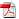Anatomy of a Misguided Maximin formulation of Info-Gap's Robustness ModelIn brief, the fatal error in Davidovitch and Ben-Haim's (2008) analysis is the uncalled for assumption that in Minimax/Maximin modeling the parameter "alpha" of the Info-Gap model must be treated as a fixed number. There are other serious Maximin/Minimax modeling errors in Davidovitch and Ben-Haim (2008).

In any case, the picture is this:Similar erroneous Maximin/Minimax formulations of Info-Gap's robustness model can be found in the following publications of the Norges Bank. For your convenience I include the abstracts as well. The full papers are available on line:

• Monetary policy under uncertainty: Min-max vs robust-satisficing strategies
by Yakov Ben-Haim, Q. Farooq Akram and Øyvind Eitrheim
Working Paper 2007/6.
Abstract:
We study monetary policy under uncertainty. A policy which ameliorates a worst case may differ from a policy which maximizes robustness and satisfices the performance. The former strategy is min-maxing and the latter strategy is robust-satisficing. We show an "observational equivalence" between robust-satisficing and min-maxing. However, there remains a "behavioral difference" between robust-satisficing and min-maxing. Policy makers often wish to respect specified bounds on target variables. The robust-satisficing policy can be more (and is never less) robust, and hence more dependable, than the min-max policy. We illustrate this in an empirical example where monetary policy making amounts to selecting the coefficients of a Taylor-type interest rate rule, subject to uncertainty in the persistence of shocks to inflation.
• Robust-satisficing monetary policy under parameter uncertainty
by Q. Farooq Akram, Yakov Ben-Haim and Øyvind Eitrheim
Working Paper 2007/14.
Abstract:
We employ the robust-satisficing approach to derive robust monetary policy when parameters of a macro model are uncertain. There is a trade-off between robustness of policies and their performance. Hence, under uncertainty, the policy maker is assumed to be content with policy performance at some satisfactory level rather than a level thought to be optimal based on available information. Our empirical analysis illustrates key properties of robust satisficing policies and compares them with min-max policies implied by the robust-control approach. Intuitively, our empirical results suggest that higher robustness can be achieved by overstating challenges to the economy and understating the abilities to meet them. How much to overstate the challenges or understate the abilities depends on the robustness sought. Robustness is achieved by lowering one's aspirations regarding the performance of policies and is therefore costly. Moreover, costs of robustness increase with the level of robustness, making robustness to apparently extreme parameter values particularly costly. We also find that robust-satisficing policies are generally less aggressive than min-max policies.

" ... Info-gap robust-satisficing is motivated by the same perception of uncertainty which motivates the min-max class of strategies: lack of reliable probability distributions and the potential for severe and extreme events. We will see that the robust-satisficing decision will sometimes coincide with a min-max decision. On the other hand we will identify some fundamental distinctions between the min-max and the robust-satisficing strategies and we will see that they do not always lead to the same decision.

First of all, if a worst case or maximal uncertainty is unknown, then the min-max strategy cannot be implemented. That is, the min-max approach requires a specific piece of knowledge about the real world: "What is the greatest possible error of the analyst's model?". This is an ontological question: relating to the state of the real world. In contrast, the robust-satisficing strategy does not require knowledge of the greatest possible error of the analyst's model. The robust-satisficing strategy centers on the vulnerability of the analyst's knowledge by asking: "How wrong can the analyst be, and the decision still yields acceptable outcomes?" The answer to this question reveals nothing about how wrong the analyst in fact is. The answer to this question is the info-gap robustness function, while the true maximal error may or may not exceed the info-gap robust satisficing. This is an epistemic question, relating to the analyst's knowledge, positing nothing about how good that knowledge actually is. The epistemic question relates to the analyst's knowledge, while the ontological question relates to the relation between that knowledge and the state of the world. In summary, knowledge of a worst case is necessary for the min-max approach, but not necessary for the robust-satisficing approach.

The second consideration is that the min-max approaches depend on what tends to be the least reliable part of our knowledge about the uncertainty. Under Knightian uncertainty we do not know the probability distribution of the uncertain entities. We may be unsure what are typical occurrences, and the systematics of extreme events are even less clear. Nonetheless the min-max decision hinges on ameliorating what is supposed to be a worst case. This supposition may be substantially wrong, so the min-max strategy may be mis-directed.

A third point of comparison is that min-max aims to ameliorate a worst case, without worrying about whether an adequate or required outcome is achieved. This strategy is motivated by severe uncertainty which suggests that catastrophic outcomes are possible, in conjunction with a precautionary attitude which stresses preventing disaster. The robust-satisficing strategy acknowledges unbounded uncertainty, but also incorporates the outcome requirements of the analyst. The choice between the two strategies — min-max and robust-satisficing — hinges on the priorities and preferences of the analyst.

The fourth distinction between the min-max and robust-satisficing approaches is that they need not lead to the same decision, even starting with the same information. ..."

Confidence in Monetary Policy
Yakov Ben-Haim and Maria Demertzis
DNB Working Paper 192, 2008.
pp. 17-18

What a mess!

My point is then that the authors demonstrate a gross misapprehension of the modeling aspects of the Minimax/Maximin paradigm. As a result, they attribute to the Minimax paradigm "undesirable" properties that are in fact the properties of the misguided ad hoc instances of Wald's model that they constructed for this comparison.

Had they set out a proper (equivalent) Maximin formulation of their Info-Gap's robust-satisficing model, their analyses would have vanished into thin air.

Moreover, what is the point in reinventing the wheel and a faulty one at that?

This is another illustration of Info-Gap proponents discoursing at length on purported "similarities and differences" between Info-Gap's robustness model and the Maximin model while the clear proof that Info-Gap's robustness model is a simple instance of Wald's Maximin model is staring them in the face.

What a waste of time!

More details on the on-going Info-Gap/Maximin saga can be found in

The interesting material is in the associated WIKIPEDIA Discussion page.

If you wish to join the campaign or just be on my mailing list for this project, send me note.

I am now working on a short book entitled "Worst-Case Analysis for Decision Making Under Severe Uncertainty". I plan to post it here when it is ready. But do not hold your breadth, I have plenty of other more urgent tasks to complete.

However, if you are eager to read this material now, I suggest the following bits and pieces that eventually will be incorporated in the book.

 Warning: This is work in progress. I plan to update the files regularly. Make sure that you have the latest update.

More on this and related topics can be found in the pages of the Worst-Case Analysis / Maximin Campaign, Severe Uncertainty, and the Info-Gap Campaign.

## Recent Articles, Working Papers, Notes

Also, see my complete list of articles

## Recent Lectures, Seminars, Presentations

If your organization is promoting Info-Gap, I suggest that you invite me for a seminar at your place. I promise to deliver a lively, informative, entertaining and convincing presentation explaining why it is not a good idea to use — let alone promote — Info-Gap as a decision-making tool.

Here is a list of relevant lectures/seminars on this topic that I gave in the last two years.

Disclaimer: This page, its contents and style, are the responsibility of the author (Moshe Sniedovich) and do not represent the views, policies or opinions of the organizations he is associated/affiliated with.